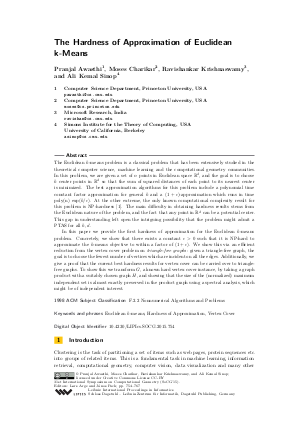Document# The Hardness of Approximation of Euclidean k-Means

### Authors Pranjal Awasthi, Moses Charikar, Ravishankar Krishnaswamy, Ali Kemal Sinop## File

LIPIcs.SOCG.2015.754.pdf
• Filesize: 0.51 MB
• 14 pages

## Cite As

Pranjal Awasthi, Moses Charikar, Ravishankar Krishnaswamy, and Ali Kemal Sinop. The Hardness of Approximation of Euclidean k-Means. In 31st International Symposium on Computational Geometry (SoCG 2015). Leibniz International Proceedings in Informatics (LIPIcs), Volume 34, pp. 754-767, Schloss Dagstuhl - Leibniz-Zentrum für Informatik (2015)
https://doi.org/10.4230/LIPIcs.SOCG.2015.754

## Abstract

The Euclidean k-means problem is a classical problem that has been extensively studied in the theoretical computer science, machine learning and the computational geometry communities. In this problem, we are given a set of n points in Euclidean space R^d, and the goal is to choose k center points in R^d so that the sum of squared distances of each point to its nearest center is minimized. The best approximation algorithms for this problem include a polynomial time constant factor approximation for general k and a (1+c)-approximation which runs in time poly(n) exp(k/c). At the other extreme, the only known computational complexity result for this problem is NP-hardness [Aloise et al.'09]. The main difficulty in obtaining hardness results stems from the Euclidean nature of the problem, and the fact that any point in R^d can be a potential center. This gap in understanding left open the intriguing possibility that the problem might admit a PTAS for all k, d. In this paper we provide the first hardness of approximation for the Euclidean k-means problem. Concretely, we show that there exists a constant c > 0 such that it is NP-hard to approximate the k-means objective to within a factor of (1+c). We show this via an efficient reduction from the vertex cover problem on triangle-free graphs: given a triangle-free graph, the goal is to choose the fewest number of vertices which are incident on all the edges. Additionally, we give a proof that the current best hardness results for vertex cover can be carried over to triangle-free graphs. To show this we transform G, a known hard vertex cover instance, by taking a graph product with a suitably chosen graph H, and showing that the size of the (normalized) maximum independent set is almost exactly preserved in the product graph using a spectral analysis, which might be of independent interest.
##### Keywords
• Euclidean k-means
• Hardness of Approximation
• Vertex Cover

## Metrics

• Access Statistics
• Total Accesses (updated on a weekly basis)
0